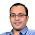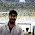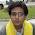### Quick Probability Questions for Interviews

1) If cor(a,b)=0.5 and cor(a,c)=0.0, what is the range for cor(b,c)

2) A deck of pokers. Three choices: A: 26 black, 26 Red; B: 13 black, 13 Red; C: random 26 card from the deck. Take the first two cards, if same color, the win $1, otherwise lose$1. Which deck is best for you if you are playing? Why?

3) What is the probability of a random generator generating 10 consecutive numbers in ascending order (assume it is a perfect random generator)

Update (24/12/2012):
Solution posted by AH in comments! Solution explained in detail by me. Thanks

1.1) It will be like solving matrix
[\sigma_a^2 0.5\sigma_a\sigma_b 0 \\
0.5\sigma_a\sigma_b \sigma_b^2 cor(b,c)\sigma_a\sigma_b \\
0 cor(b,c)\sigma_a\sigma_b \sigma_c^2]
such that each row and column sum is 1

Was not able to solve it completely

2) 26B 26R Probability = 25/51
13R13B 12/25
26 random number
Let x R and 26-x B cards are choosen
Now x can be uniformly between 0 to 26
Probability = \integral_{0}{26} (x(x-1)+(26-x)(25-x))/(26*25*26) dx
Probability = 0.653
Deck 3 is the best

2.1.) Correlation matrix should be non negative definite => determinants at all levels should be >= 0 => x^2 < 3/4

2.) Expected Winnings:
Choice A: ((1)*(25/51)) + ((-1)*(26/51)) = -1/51
Choice B: ((1)*(12/25)) + ((-1)*(13/25)) = -1/13
Choice C: Same as A (Sampling 26 random cards and then taking the first two is same as just taking the first two from the whole deck)

3.) 1/10!

3.@AH.. Thanks for the correct solutions to all the three problems.

Q.1

A more detailed solution of first question:
The correlation matrix for a, b, c is as follows:
first row as (1.0, 0.5, 0.0)
second row as (0.5, 1.0, x)
third row as (0.0, x, 1.0)

has to be positive definite
Hence, 1*(1-x^2) - 0.5*(0.5) >= 0
Hence, x^2 < 0.75

Q.2

A more mathematical explanation for the Choice C of the second question is "Total Probability Theorem".

1.Q1) why is variance of a, b, c =1

2.without loss of generality you can take mean=0 and variance=1. This is because correlation is invariant over location and scale transformations. Correlation between (x,y) =
Correlation between ((x-a)/b , (y-c)/d)